Thank you for visiting nature.com. You are using a browser version with limited support for CSS. To obtain the best experience, we recommend you use a more up to date browser (or turn off compatibility mode in Internet Explorer). In the meantime, to ensure continued support, we are displaying the site without styles and JavaScript.

# Control of the spin geometric phase in semiconductor quantum rings

## Abstract

Since the formulation of the geometric phase by Berry, its relevance has been demonstrated in a large variety of physical systems. However, a geometric phase of the most fundamental spin-1/2 system, the electron spin, has not been observed directly and controlled independently from dynamical phases. Here we report experimental evidence on the manipulation of an electron spin through a purely geometric effect in an InGaAs-based quantum ring with Rashba spin-orbit coupling. By applying an in-plane magnetic field, a phase shift of the Aharonov–Casher interference pattern towards the small spin-orbit-coupling regions is observed. A perturbation theory for a one-dimensional Rashba ring under small in-plane fields reveals that the phase shift originates exclusively from the modulation of a pure geometric-phase component of the electron spin beyond the adiabatic limit, independently from dynamical phases. The phase shift is well reproduced by implementing two independent approaches, that is, perturbation theory and non-perturbative transport simulations.

## Introduction

Geometric phases arise in wave systems1 where the parameters of the wave function are cycled around a circuit. Such phase factors can be observed via interference of waves traversing different paths. Berry1 has shown that the electron spin wave function in an adiabatic evolution acquires a geometric phase that depends only on the geometry of the traversed path in the parameter space. As a consequence, the geometric phase is robust against dephasing. This is in contrast to the time-dependent dynamical phase of a particle2,3,4. Experimentally, geometric-phase factors have been observed in various systems5,6. However, the geometric phase of an electronic spin has never been directly observed and manipulated independently of the dynamical phase before.

Here we demonstrate the control of the geometric phase of an electron spin in a mesoscopic semiconductor device in which an array of rings forms interference paths. The experiment shows geometric phases beyond the adiabatic limit7. A geometric-phase shift of the Aharonov–Casher (AC) effect8,9,10 is induced with an in-plane magnetic field and measured in an interference pattern of the electron current. Our findings show manipulation of the geometric phase independently of the dynamical phase without introducing additional geometric-phase factors such as the Aharonov–Bohm phase.

## Results

### Concept of present experiment

Spins in a magnetic field acquire a dynamical phase, which physically corresponds to phase accumulation because of the spin precession around the magnetic field. In a mesoscopic ring system with an inhomogeneous magnetic field, the spins acquire also a geometric phase that depends on the path of the spin. As an example, consider a one-dimensional (1D) ring in a textured magnetic field11 as shown in Fig. 1a. The magnetic field seen by a propagating electron along the ring subtends, from its rest frame, a solid angle in the magnetic-field space. The spin acquires not only a dynamical phase but also a geometric phase, which is proportional to the solid angle (Fig. 1b).Figure 1: Schematic illustration of spin geometric phases in quantum rings.

The Bychkov–Rashba spin-orbit (SO) coupling12 can be used to create an effective in-plane magnetic-field texture (Fig. 1c). The coupling is induced at an interface between semiconductor layers having different energy gaps by an electric field perpendicular to the layers (say along the z axis). It is a relativistic effect where an electron travelling through an electric field feels an effective SO magnetic field BSO, the direction of which is perpendicular both to the electric field and a wave vector k, that is, BSOαR(k × ez). Here the electric field enters through the Rashba SO-coupling constant αR that is therefore electrically tunable with a top-gate electrode13,14, and ez denotes a unit vector in z direction. This effective Rashba field couples to the electron spin, which acquires dynamical and geometric phases in the transport around the ring15,16,17. Interference of these spin-related phases gives rise to conductance oscillations when αR is varied18,19,20. Recently, the SO-induced geometric phase has been extracted21 from a detailed analysis of spin-interference effects as a function of the SO-coupling constant αR and the ring radius r.

In a 1D Rashba ring placed in the xy plane, a travelling electron has a non-zero wave vector k only in the in-plane direction because of the electrostatic confinement along ez. Consequently, the effective field BSO seen by the electron always points in the radial direction (see Fig. 1c). However, if the revolution frequency ω0 around the ring is comparable to the spin-precession frequency ωSO, spin transport is non-adiabatic. As a result the electron spin does not exactly precess around BSO (see refs 22, 23); spin eigenstates are no longer parallel to BSO and the corresponding polar angle, θ, satisfies, with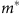being the effective mass of an electron and ħ the reduced Planck’s constant, as if there was an effective magnetic field normal to the ring’s plane besides BSO as depicted in Fig. 1d. This is in analogy to NMR experiment where an rf magnetic field yields a static out-of-plane field in a rotating frame of reference. Hence, an electron spin in the Rashba ring acquires a geometric phase, which corresponds to the solid angle subtended by the spin eigenstates.

Now, consider a Rashba ring with an external magnetic field applied parallel to the ring plane as shown in Fig. 1e: the total magnetic field seen by the electron is uniformly tilted towards the applied field direction, which changes the solid angle in the magnetic-field space (Fig. 1f). As we will show, this allows for controlled modulation of the pure geometric phase independently of the dynamical phase. We emphasize that the separation of the geometric and dynamical phases has not been achieved in mesoscopic spin systems so far: the SO-coupling constant αR and the ring radius r modulate both the geometric and dynamical phases simultaneously through the degree of adiabaticity, QR.

### In-plane field dependence of the AC effect

In our experiment, an array of 40 × 40 rings (Fig. 2a) and a Hall bar have been fabricated lithographically from a shallow InGaAs quantum well (see ref. 24 for the basic properties). The Hall bar and the ring array are in the same current path and they have been covered with the same top-gate electrode. The electrical resistance has been measured with a standard ac lock-in technique at a temperature of 1.5 K with a liquid helium cryostat where we can apply magnetic fields both perpendicular and parallel to the ring plane. Figure 2b shows the Altshuler–Aronov–Spivak (AAS) effect in the ring array at a gate voltage of 1.1V as a function of the perpendicular magnetic-field strength.Figure 2: Altshuler–Aronov–Spivak and AC oscillations in an InGaAs-based ring array.

We study the control of the spin geometric phase via the AC effect8,9,10, the electromagnetic dual of the Aharonov–Bohm effect. Specifically, in our system, the AC effect is realized as the gate modulation of spin interference. The AAS amplitude oscillates with the gate voltage, as shown in Fig. 2c, indicating the gate modulation of the spin phase. This oscillatory behaviour of the magnetoresistance at zero (perpendicular) external field is visualized in Fig. 2d. Because of disorder in the samples and the ensemble average over the ring array, pairs of time-reversed (TR) paths, that is, the path pairs travelling in opposite directions around the ring, contribute predominantly to the interference pattern in the magnetoresistance. The AC effect in the TR paths gives rise to the resistance modulation given by25,23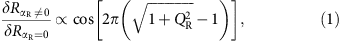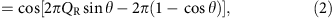where $\text{δ}{R}_{{\alpha }_{\text{R}}\ne 0}$ and $\text{δ}{R}_{{\alpha }_{\text{R}}=0}$ are, respectively, the electrical resistance with and without Rashba SO coupling in a ring. Equation (2), plotted as the red dashed line in Fig. 2d, is in agreement with experiment. The phase of the cosine function in equation (2) shows that the AC phase comprises two components, namely, a dynamical component 2πQR sin θ and a geometric one −2π(1−cos θ). The latter exactly represents the solid angle subtended by the spin eigenstates in the Rashba ring (Fig. 1d).

The dependence of the AC effect on the in-plane magnetic fieldin experiment is shown in Fig. 3a,b. In Fig. 3a, the gate voltage is translated to the Rashba SO-coupling constant αR by using the relation obtained from the Shubnikov–de Haas analysis13 in the Hall bar. Asis applied, the amplitude of the AC effect is suppressed because of spin-induced TR symmetry breaking26 (see the Supplementary Note 1). Further, the AC oscillations exhibit a quadratic shift withtowards positive gate voltages, that is, weaker Rashba SO-coupling strengths. In the following, we explain the observed shift by the sole modulation of the geometric phase as shown in Fig. 1f.Figure 3: Geometric-phase shift because of an in-plane magnetic field .

### Perturbation theory and numerical transport calculations

To evaluate the effect of in-plane magnetic fields on the geometric phases in the limit of small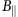, we employ perturbation theory for a 1D Rashba ring (see the Methods section). The phase shift is calculated to first order in. We find that the AC interference between TR pairs of paths leads to conductance oscillations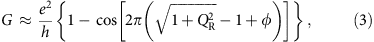where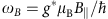and. Further, kF is the Fermi wave number,is the Landé g-factor and μB is the Bohr magneton. By comparing equations (1) and (3), we find thatprovides an additional phase 2π φ quadratic in. As plotted in Fig. 3c,d, equation (3) reproduces the observed phase shift within the energy range of small Zeeman energy (due to) compared with the kinetic and Rashba SO-coupling energies. A similar quadratic shift in the interference peak positions has been recently calculated also for weakly coupled rings27.

To determine the origin of the AC-phase shift, we calculate a geometric-phase contribution φAA using eigenstatescalculated to first order in the in-plane field:with ϕ being the azimuthal angle in the ring plane and j being an integer. By comparing equations (1, 2, 3) and (6), we confirm that the perturbation term φ has a purely geometric origin, at least to first order in the in-plane field strength. This is because electron spins in the TR interference paths experience a uniaxialfield, which does not yield a dynamical phase difference through the spin precession. Hence, our central result is that the observed shift of the AC phase is induced by the modulation of the pure geometric phase of an electron spin.

Note that the wave number enters into the denominator of the geometric-phase shift term φ, equation (4). In our samples the conducting channel contains at least six transverse modes, each having a unique wave number. To take into account the effect of the multiple transport modes and for an adequate treatment offield strengths beyond the perturbative limit, we employ the numerical recursive Green’s function method28 (see the Supplementary Note 2). Results calculated at fixed carrier density 1.0 × 1016 m−2 are shown in Fig. 3e,f. The geometric shift of the AC oscillations occurs even in the presence of multiple modes (see the Supplementary Note 3).

## Discussion

Figure 4 shows the comparison of the geometric shift in experiment (filled symbols) with the perturbation theory (solid lines) and the non-perturbative numerical calculations (open symbols) at peak and dip positions of the AC oscillations (P1–P4; see Fig. 3). The carrier density in the numerical calculations is increased with gate voltage (see the Supplementary Note 2). The perturbation theory for a single transverse mode is in good agreement with experiment in the regime of strong SO coupling (P1 and P2). This indicates that the phase shift is dominated only by a few modes or just the lowest one, presumably because of stronger decoherence for higher transverse modes, which have the smaller wave numbers along the ring. The discrepancy is significant at weak SO-coupling regions (P3 and P4) that are beyond the limit of validity of the perturbation expansion. We note that wave numbers associated with the modes in multimode numerical calculations depend on the energy level spacing in the confining channel of the ring. Numerical calculations assume a square-shaped potential, which may lead to the overestimation of the phase shift at P1. Further, in the numerical model a transition from weak antilocalization to weak localization with changing Rashba SO coupling results in the increased background resistance at small SO fields (see Fig. 3f). As a result, the positions of P3 and P4 may shift. However, almost all the results show the peaks and dips shift towards weak Rashba SO coupling, that is, positive gate voltages. We are thus able to reasonably simulate and explain the experimentally observed spin-phase shift by both numerical calculations and the perturbation theory.Figure 4: Geometrical shift of peak and dip positions of the AC oscillations with an in-plane field .

For further evidence, we have measured the radius dependence of the-induced phase shift. Figure 5 shows the comparison of the shift in samples of rings with r=0.6 and 1.1 μm. We see that both in experiment and in the perturbation theory, geometric-phase shift increases with the ring radius. This confirms an important prediction of our theory contained in the equations (3) and (4). From the comparison between experiment and the theory, the diamagnetic shift of energy is negligible in our samples because the diamagnetic effect should not have the radius dependence. To the best of our knowledge, this is the first experimental demonstration of the pure geometric-phase control of an electron spin in solid-state devices. We conclude that this multiparameter control of the spin geometric phase may pave the way for future spintronic applications, such as geometric-gate operation29 of a solid-state flying qubit or control of persistent spin currents in a mesoscopic ring11,30, which may have potential applications for non-volatile memory devices.

## Methods

### Sample fabrication and measurements

The sample was epitaxially grown on a (001) InP substrate by metal organic chemical vapour deposition. It consists of, from the bottom, In0.52Al0.48As (200 nm, buffer layer)/In0.52Al0.48As (6 nm, carrier supply layer; Si-doping concentration of 4 × 1018 m−3)/In0.52Al0.48As (15 nm, spacer layer)/In0.53Ga0.47As (2.5 nm, quantum well)/In0.70Ga0.30As (10 nm, quantum well)/In0.53Ga0.47As (2.5 nm, quantum well)/InP (5 nm, stopper layer)/In0.52Al0.48As (20 nm, barrier layer)/AlAs (1.5 nm, barrier layer)/In0.52Al0.48As (5 nm, cap layer). An array of 40 × 40 rings and a Hall bar (70 × 280 μm2) have been fabricated by means of e-beam lithography and reactive-ion etching. To form an ohmic contact, AuGeNi was used (annealing temperature of 275 °C, 7 min). As a top-gate insulator, a 200-nm-thick Al2O3 layer was deposited by atomic layer deposition. A Cr/Au top-gate electrode was fabricated on the insulator layer.

A four-terminal standard lock-in technique has been used to measure the perpendicular magnetoresistance of the ring array as a function of the gate voltage and the in-plane magnetic field. At a given gate voltage, the Rashba SO-coupling constant and the carrier density in the ring structure can be estimated by measuring the Shubnikov–de Haas oscillations in the Hall bar.

### Perturbation theory

We introduce a perturbative approach for studying the effect of small in-plane magnetic fields in the conductance of 1D rings subject to Rashba SO coupling. We demonstrate that the in-plane field acts on the geometrical spin phase alone without contributing to dynamical phases at the lowest perturbative order, allowing the controlled manipulation of geometrical phases. The Hamiltonian readswith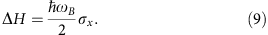Here H0 is the unperturbed Hamiltonian of a 1D Rashba ring of radius r lying in the xy plane parametrized by the azimuthal angle ϕ. We have defined ω0=ħ/mr2 and ωSO=2αRr as the characteristic frequencies of the kinetic and SO Rashba terms, respectively, together with the polar Pauli matrices σr=cosϕ σx+sinϕ σy and σϕ=−sinϕ σx+cosϕ σy. The third term in equation (8) guarantees H0 to be Hermitian22, losing relevance for large momenta. The ΔH in equation (9) is a perturbation on H0 by an in-plane magnetic fieldalong the x direction, withthe corresponding Larmor frequency. The unperturbed eigenstates |n, λ, s0 of H0 (with spin s=↑,↓, travel sense λ=±1, and orbital wave numbers n≥0) are23where θ is the inclination of the local spin-quantization axis with respect to the z axis given by tanθ=ωSO/ω0QR. Notice that θ does not depend on any quantum number, being shared by all eigenstates. The corresponding eigenenergies areWhere ↑ spins are defined to maximize the eigenenergies. We notice that states with opposite travel sense |n,−,s0 and |n−1,+,s0 are degenerated (that is,). In addition, we find that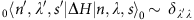, namely, the perturbation ΔH does not mix degenerate states. This means that we can apply a non-degenerate perturbation theory. We further notice that an additional degeneracy (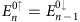) arises in the unperturbed system for vanishing Rashba coupling (QR=0), meaning that the perturbative approach we present here is not valid for.

We calculate the perturbed eigenstates |n,λ,s› and eigenenergiesto the lowest order in ωB. Taking into account that 0n±1, λ, sH|n, λ, s0=λs(ħωB/4)sinθ and 0n±1, λ, −sH|n, λ, s0ωB(cosθ±s)/4, we find (by following, for example, the textbook by Sakurai31)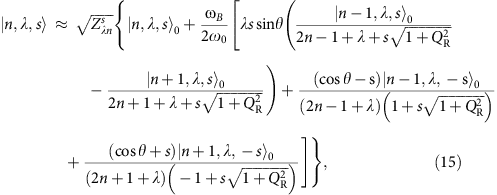with normalization constant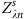, and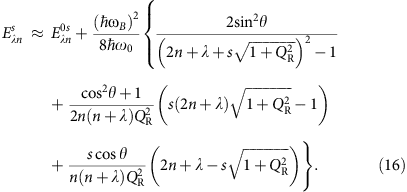We calculate the ring conductance by following the procedure introduced in (refs 23, 25). This is based on the 1D Landauer formula, where the conductance G is determined by the quantum probabilities of transmission Tσσ′=|tσσ′|2 (with amplitudes tσσ′) for incoming (outgoing) spins σ′ (σ). We consider strongly coupled contacts at diametrically opposed locations ϕ=0 and ϕ=π. Because of the energy splitting, incoming spin carriers with Fermi energy EF entering at ϕ=0 can propagate coherently through the ring along four possible channels with (non-integer) wave numbersdepending on spin (s) and travel sense (λ). Theare determined by solvingin equation (16) and the relative weight of each propagation channel is given by the projection of the incoming spin state on the corresponding spin eigenstates, equation (15), at the entrance point. We assume that, because of strong coupling to the contacts, the carriers propagate directly from the entrance (ϕ=0) to the exit (ϕ=π) point along the shortest paths without any additional winding (see Fig. 6a). After interference, we findby summing over all spin indices, with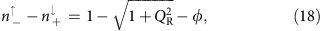where we assumed a large electron density corresponding toin equations (15) and (16), in agreement with the experimental conditions. The conductance then readsThe result of equation (20) holds for single ballistic rings. For an ensemble of rings subject to shape fluctuations and/or disorder, instead, the main contribution to the sample-averaged conductance comes from TR paths leading to the AAS oscillations (see Fig. 6b). By following a similar procedure (taking into account that TR paths contribute to the transmission probability T through the reflection probability R by unitarity T+R=1), we find an AAS conductance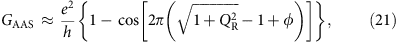which applies to the reported experiment. The φ of equation (19) corresponds to a phase shift in the AC conductance introduced by the in-plane magnetic field. It is quadratic in the field’s strength ωB and proportional to. The latter illustrates the known fact that spin dynamics under Zeeman coupling depends on the electrons velocity32, in contrast to Rashba coupling. We further notice that φ is an increasing function of the ring’s radius r.

To demonstrate the geometric nature of φ, we first notice in equations (20) and (21) that, whereandare the unperturbed contributions to the dynamical and geometrical phases, correspondingly, with Ω0 the solid angle described by the unperturbed spin eigenstates over the ring (see ref. 23). The limit of adiabatic spin dynamics, where purely geometrical Berry phases arise1, corresponds to QR→∞(θ→π/2). For a finite QR (0<θ<π/2), the spin dynamics is non-adiabatic and the geometrical phase (depending explicitly on QR) is referred to as the Aharonov–Anandan phase7. For the perturbed eigenstates, by defining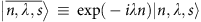, the geometric-phase contribution φAA can be extracted as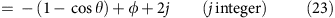There we find that the magnetic shift φ is purely geometric. This means that, at the lowest order of perturbation, the in-plane magnetic field modifies the eigenstate spin texture without contributing to the dynamical spin phase. More explicitly, from equation (24) we find ΔΩ=−2π φ showing that the in-plane field perturbs the spin eigenstates by reducing the solid angle with respect to the unperturbed case. This means that the perturbation hinders adiabatic spin dynamics: In the regime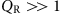(with unperturbed spinors along θπ/2) spins are lifted from the ring’s plane by the in-plane magnetic field. The full Aharonov–Anandan geometric phase in the perturbed system reads (mod 2π) γ=−π[(1−cosθ)−ϕ] for single ballistic rings (Fig. 6a) and γ=−2π[(1−cosθ)−ϕ] for rings ensembles in AAS configuration (Fig. 6b).

How to cite this article: Nagasawa, F. et al. Control of the spin geometric phase in a semiconductor quantum ring. Nat. Commun. 4:2526 doi: 10.1038/ncomms3526 (2013).

## References

1. Berry, M. V. Quantal phase factors accompanying adiabatic changes. Proc. R. Soc. Lond. A 392, 45–57 (1984).

2. Carollo, A., Fuentes-Guridi, I., Santos, M. F. & Vedral, V. Geometric phase in open systems. Phys. Rev. Lett. 90, 160402 (2003).

3. Zhu, S.-L. & Zanardi, P. Geometric quantum gates that are robust against stochastic control errors. Phys. Rev. A 72, 020301 (2005).

4. Filipp, S. et al. Experimental demonstration of the stability of Berry's phase for a spin-1/2 particle. Phys. Rev. Lett. 102, 030404 (2009).

5. Tomita, A. & Chiao, R. Y. Observation of Berry's topological phase by use of an optical fiber. Phys. Rev. Lett. 57, 937–940 (1986).

6. Leek, P. J. et al. Observation of Berry's phase in a solid-state qubit. Science 318, 1889–1892 (2007).

7. Aharonov, Y. & Anandan, J. Phase change during a cyclic quantum evolution. Phys. Rev. Lett. 58, 1593–1596 (1987).

8. Aharonov, Y. & Casher, A. Topological quantum effects for neutral particles. Phys. Rev. Lett. 53, 319–321 (1984).

9. Meir, Y., Gefen, Y. & Entin-Wohlman, O. Universal effects of spin-orbit scattering in mesoscopic systems. Phys. Rev. Lett. 63, 798–800 (1989).

10. Mathur, A. & Stone, A. D. Quantum transport and the electronic Aharonov-Casher effect. Phys. Rev. Lett. 68, 2964–2967 (1992).

11. Loss, D., Goldbart, P. & Balatsky, A. V. Berry's phase and persistent charge and spin currents in textured mesoscopic rings. Phys. Rev. Lett. 65, 1655–1658 (1990).

12. Bychkov, Y. A. & Rashba, E. I. Oscillatory effects and the magnetic susceptibility of carriers in inversion layers. J. Phys. C 17, 6039–6045 (1984).

13. Nitta, J., Akazaki, T., Takayanagi, H. & Enoki, T. Gate control of spin-orbit interaction in an inverted In0.53Ga0.47As/In0.52Al0.48As Heterostructure. Phys. Rev. Lett. 78, 1335–1338 (1997).

14. Engels, G., Lange, J., Schäpers, T. & Lüth, H. Experimental and theoretical approach to spin splitting in modulation-doped InxGa1−xAs/InP quantum wells for B→0. Phys. Rev. B 55, R1958–R1961 (1997).

15. Aronov, A. G. & Lyanda-Geller, Y. B. Spin-orbit Berry phase in conducting rings. Phys. Rev. Lett. 70, 343–346 (1993).

16. Morpurgo, A. F., Heida, J. P., Klapwijk, T. M., van Wees, B. J. & Borghs, G. Ensemble-average spectrum of Aharonov-Bohm conductance oscillations: evidence for spin-orbit-induced Berry's phase. Phys. Rev. Lett. 80, 1050–1053 (1998).

17. Yau, J.-B., De Poortere, E. P. & Shayegan, M. Aharonov-Bohm oscillations with spin: Evidence for Berry's phase. Phys. Rev. Lett. 88, 146801 (2002).

18. Bergsten, T., Kobayashi, T., Sekine, Y. & Nitta, J. Experimental demonstration of the time reversal Aharonov-Casher effect. Phys. Rev. Lett. 97, 196803 (2006).

19. König, M. et al. Direct observation of the Aharonov-Casher phase. Phys. Rev. Lett. 96, 076804 (2006).

20. Grbić, B. et al. Aharonov-Bohm oscillations in the presence of strong spin-orbit interactions. Phys. Rev. Lett. 99, 176803 (2007).

21. Nagasawa, F., Takagi, J., Kunihashi, Y., Kohda, M. & Nitta, J. Experimental demonstration of spin geometric phase: radius dependence of time-reversal Aharonov-Casher oscillations. Phys. Rev. Lett. 108, 086801 (2012).

22. Meijer, F. E., Morpurgo, A. F. & Klapwijk, T. M. One-dimensional ring in the presence of Rashba spin-orbit interaction: derivation of the correct Hamiltonian. Phys. Rev. B 66, 033107 (2002).

23. Frustaglia, D. & Richter, K. Spin interference effects in ring conductors subject to Rashba coupling. Phys. Rev. B 69, 235310 (2004).

24. Kunihashi, Y., Kohda, M. & Nitta, J. Enhancement of spin lifetime in gate-fitted InGaAs narrow wires. Phys. Rev. Lett. 102, 226601 (2009).

25. Nitta, J., Meijer, F. E. & Takayanagi, H. Spin-interference device. Appl. Phys. Lett. 75, 695–697 (1999).

26. Meijer, F. E., Morpurgo, A. F., Klapwijk, T. M., Koga, T. & Nitta, J. Competition between spin-orbit interaction and Zeeman coupling in Rashba two-dimensional electron gases. Phys. Rev. B 70, 201307 (2004).

27. Joibari, F. K., Blanter, Y. M. & Bauer, G. E. W. Aharonov-Casher effect in quantum ring ensemblesPhys. Rev. B 88, 115410 (2013).

28. Wimmer, M. & Richter, K. Optimal block-tridiagonalization of matrices for coherent charge transport. J. Comput. Phys. 228, 8548–8565 (2009).

29. Ichikawa, T., Bando, M., Kondo, Y. & Nakahara, M. Geometric aspects of composite pulses. Phil. Trans. R. Soc. A 370, 4671–4689 (2012).

30. Splettstoesser, J., Governale, M. & Zülicke, U. Persistent current in ballistic mesoscopic rings with Rashba spin-orbit coupling. Phys. Rev. B 68, 165341 (2003).

31. Sakurai, J. J. Modern Quantum Mechanics Ch. 5.1 (Addison Wesley (1993).

32. Popp, M., Frustaglia, D. & Richter, K. Conditions for adiabatic spin transport in disordered systems. Phys. Rev. B 68, 041303(R) (2003).

## Acknowledgements

We acknowledge support from Strategic Japanese-German Joint Research Program. H.S. and K.R. thank DFG for support within Research Unit FOR 1483. D.F. acknowledges support from the Ramón y Cajal program, from the Spanish Ministry of Science’s project No. FIS2011-29400, and from the Junta de Andaluca’s Excellence Project No. P07-FQM-3037. F.N. and J.N. are grateful to M.Kohda for valuable discussions. J.N. thanks N.Nagaosa for valuable discussions. This work was financially supported by Grants-in-Aid from the Japan Society for the Promotion of Science (JSPS) No. 22226001.

## Author information

Authors

### Contributions

F.N. performed measurements, analysed the results and wrote the manuscript. D.F. derived the theoretical model and wrote part of the manuscript. H.S. performed the numerical calculations and analysed them with K.R. H.S. wrote part of the Supplementary Information. All authors discussed the results and worked on the manuscript at all stages. J.N. directed the research.

### Corresponding author

Correspondence to Junsaku Nitta.

## Ethics declarations

### Competing interests

The authors declare no competing financial interests.

## Supplementary information

### Supplementary Information

Supplementary Figures S1-S6, Supplementary Notes 1-3 and Supplementary References (PDF 161 kb)

## Rights and permissions

Reprints and Permissions

Nagasawa, F., Frustaglia, D., Saarikoski, H. et al. Control of the spin geometric phase in semiconductor quantum rings. Nat Commun 4, 2526 (2013). https://doi.org/10.1038/ncomms3526

• Accepted:

• Published:

• DOI: https://doi.org/10.1038/ncomms3526

• ### Geometric phase from Aharonov–Bohm to Pancharatnam–Berry and beyond

• Eliahu Cohen
• Hugo Larocque
• Ebrahim Karimi

Nature Reviews Physics (2019)

• ### Measurement of geometric dephasing using a superconducting qubit

• S. Berger
• M. Pechal
• S. Filipp

Nature Communications (2015)

• ### Noncyclic geometric quantum computation and preservation of entanglement for a two-qubit Ising model

• H. Rangani Jahromi
• M. Amniat-Talab

Quantum Information Processing (2015)